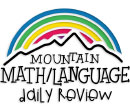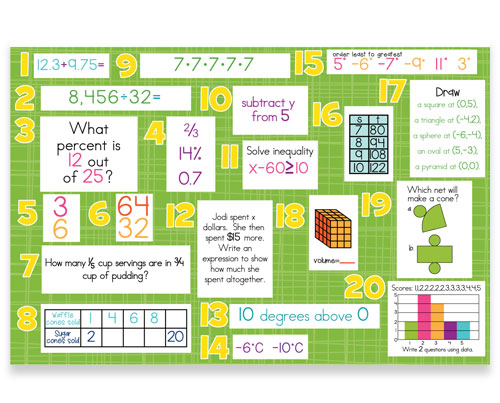WHAT YOU GET:
• 32 WEEKS OF DAILY REVIEW
• INSTRUCTIONS
• ONE MASTER STUDENT RESPONSE SHEET
• PRINTED ON HIGH-QUALITY CARD STOCK
• KNOWLEDGEABLE STAFF FOR ANY ASSISTANCE

This 6th GRADE COMMON CORE DAILY REVIEW MATH BULLETIN BOARD KIT is the DAILY REVIEW your students need to MASTER the concepts you have taught.

Research has proven that LONG-TERM MASTERY comes from SPIRAL REVIEW, also called DISTRIBUTED PRACTICE and SPACED REPETITION.

The Bulletin Board Kit is designed for total classroom viewing. It hangs on bulletin boards, walls, white boards, pocket charts, and science display boards. Once hung, it can remain displayed for the entire year. The cards can be interchanged, creating flexible and adjustable DAILY REVIEW.
1. 6.NS.B.3-Fluently add, subtract, multiply, and divide multi-digit decimals using the standard algorithm for each operation.
2. 6.NS.B.2-Fluently divide multi-digit numbers using the standard algorithm.
3. 6.RP.A.3.C-Find a percent of a quantity as a rate per 100 (e.g., 30% of a quantity means 30/100 times the quantity); solve problems involving finding the whole, given a part and the percent.
4. Converting and ordering fractions, decimals, and percentages.
5. 6.NS.B.4-Find the least common multiple of two whole numbers less than or equal to 12.
6. 6.NS.B.4-Find the greatest common factor of two whole numbers less than or equal to 100.
7. 6.NS.A.1-Interpret and compute quotients of fractions, and solve word problems involving division of fractions by fractions, e.g., by using visual fraction models and equations to represent the problem.
6.RP.A.2-Understand the concept of a unit rate a/b associated with a ratio a:b with b  0, and use rate language in the context of a ratio relationship.
6.RP.A.3-Use ratio and rate reasoning to solve real-world and mathematical problems, e.g., by reasoning about tables of equivalent ratios, tape diagrams, double number line diagrams, or equations.
8. 6.RP.A.3.A-Make tables of equivalent ratios relating quantities with whole-number measurements, find missing values in the tables, and plot the pairs of values on the coordinate plane. Use tables to compare ratios.
6.RP.A.3.B-Solve unit rate problems including those involving unit pricing and constant speed.
9. 6.EE.A.1-Write and evaluate numerical expressions involving whole-number exponents.
10. 6.EE.A.2.A-Write, read, and evaluate expressions in which letters stand for numbers.
11. 6.EE.A.3-Apply the properties of operations to generate equivalent expressions.
6.EE.B.5-Understand solving an equation or inequality as a process of answering a question: which values from a specified set, if any, make the equation or inequality true? Use substitution to determine whether a given number in a specified set makes an equation or inequality true.
12. 6.EE.B.6-Use variables to represent numbers and write expressions when solving a real-world or mathematical problem; understand that a variable can represent an unknown number, or, depending on the purpose at hand, any number in a specified set.
6.EE.B.7-Solve real-world and mathematical problems by writing and solving equations of the form x + p = q and px = q for cases in which p, q and x are all nonnegative rational numbers.
6.EE.B.8-Write an inequality of the form x > c or x < c to represent a constraint or condition in a real-world or mathematical problem. Recognize that inequalities of the form x > c or x < c have infinitely many solutions; represent solutions of such inequalities on number line diagrams.
13. 6.NS.C.5-Understand that positive and negative numbers are used together to describe quantities having opposite directions or values.
6.NS.C.7.C-Understand the absolute value of a rational number as its distance from 0 on the number line; interpret absolute value as magnitude for a positive or negative quantity in a real-world situation.
14. 6.NS.C.7.A-Interpret statements of inequality as statements about the relative position of two numbers on a number line diagram.
6.NS.C.7.B-Write, interpret, and explain statements of order for rational numbers in real-world contexts.
15. 6.NS.C.6.A-Recognize opposite signs of numbers as indicating locations on opposite sides of 0 on the number line.
6.NS.C.7.C-Understand the absolute value of a rational number as its distance from 0 on the number line; interpret absolute value as magnitude for a positive or negative quantity in a real-world situation.
6.NS.C.7.D-Distinguish comparisons of absolute value from statements about order.
16. 6.EE.C.9-Use variables to represent two quantities in a real-world problem that change in relationship to one another; write an equation to express one quantity, thought of as the dependent variable, in terms of the other quantity, thought of as the independent variable. Analyze the relationship between the dependent and independent variables using graphs and tables, and relate these to the equation.
17. 6.NS.C.6.B-Understand signs of numbers in ordered pairs as indicating locations in quadrants of the coordinate plane; recognize that when two ordered pairs differ only by signs, the locations of the points are related by reflections across one or both axes.
6.NS.C.6.C-Find and position integers and other rational numbers on a horizontal or vertical number line diagram; find and position pairs of integers and other rational numbers on a coordinate plane.
6.NS.C.8-Solve real-world and mathematical problems by graphing points in all four quadrants of the coordinate plane. Include use of coordinates and absolute value to find distances between points with the same first coordinate or the same second coordinate.
6.G.A.3-Draw polygons in the coordinate plane given coordinates for the vertices; use coordinates to find the length of a side joining points with the same first coordinate or the same second coordinate. Apply these techniques in the context of solving real-world and mathematical problems.
18. 6.G.A.1-Find the area of right triangles, other triangles, special quadrilaterals, and polygons by composing into rectangles or decomposing into triangles and other shapes; apply these techniques in the context of solving real-world and mathematical problems.
6.G.A.2-Find the volume of a right rectangular prism with fractional edge lengths by packing it with unit cubes of the appropriate unit fraction edge lengths, and show that the volume is the same as would be found by multiplying the edge lengths of the prism.
19. 6.G.A.4-Represent three-dimensional figures using nets made up of rectangles and triangles, and use the nets to find the surface area of these figures. Apply these techniques in the context of solving real-world and mathematical problems.
20. 6.SP.A.1-Recognize a statistical question as one that anticipates variability in the data related to the question and accounts for it in the answers.
6.SP.A.2-Understand that a set of data collected to answer a statistical question has a distribution which can be described by its center, spread, and overall shape.
6.SP.A.3-Recognize that a measure of center for a numerical data set summarizes all of its values with a single number, while a measure of variation describes how its values vary with a single number.
6.SP.4.B-Display numerical data in plots on a number line, including dot plots, histograms, and box plots.
6.SP.B.5-Summarize numerical data sets in relation to their context.
ONLINE REVIEW VS.
CENTER EDITION VS.
BULLETIN BOARD KIT
ONLINE REVIEW
Is projected onto an interactive white board, creating a virtual board. It can be viewed by the entire classroom. Answers can be displayed onto the board at the click of a button. Up to seven questions can be isolated and magnified.

CENTER EDITION
Is organized on a 13"x19" flip chart. It can be viewed by 5-6 students at a time. It was designed for small group review, such as rotations, scoots, centers, or math-around-the-classroom. It has an answer key, heavy-duty stand, and high-gauged coil.

BULLETIN BOARD KIT
It is designed for total classroom view. It hangs on bulletin boards, walls, white boards, pocket charts, and science display boards. Once hung, it can remain displayed for the entire year. The cards can be interchanged, creating flexible and adjustable DAILY REVIEW.

\$119.95 QTY:
Bulletin Boards   |   6th Grade Common Core Math